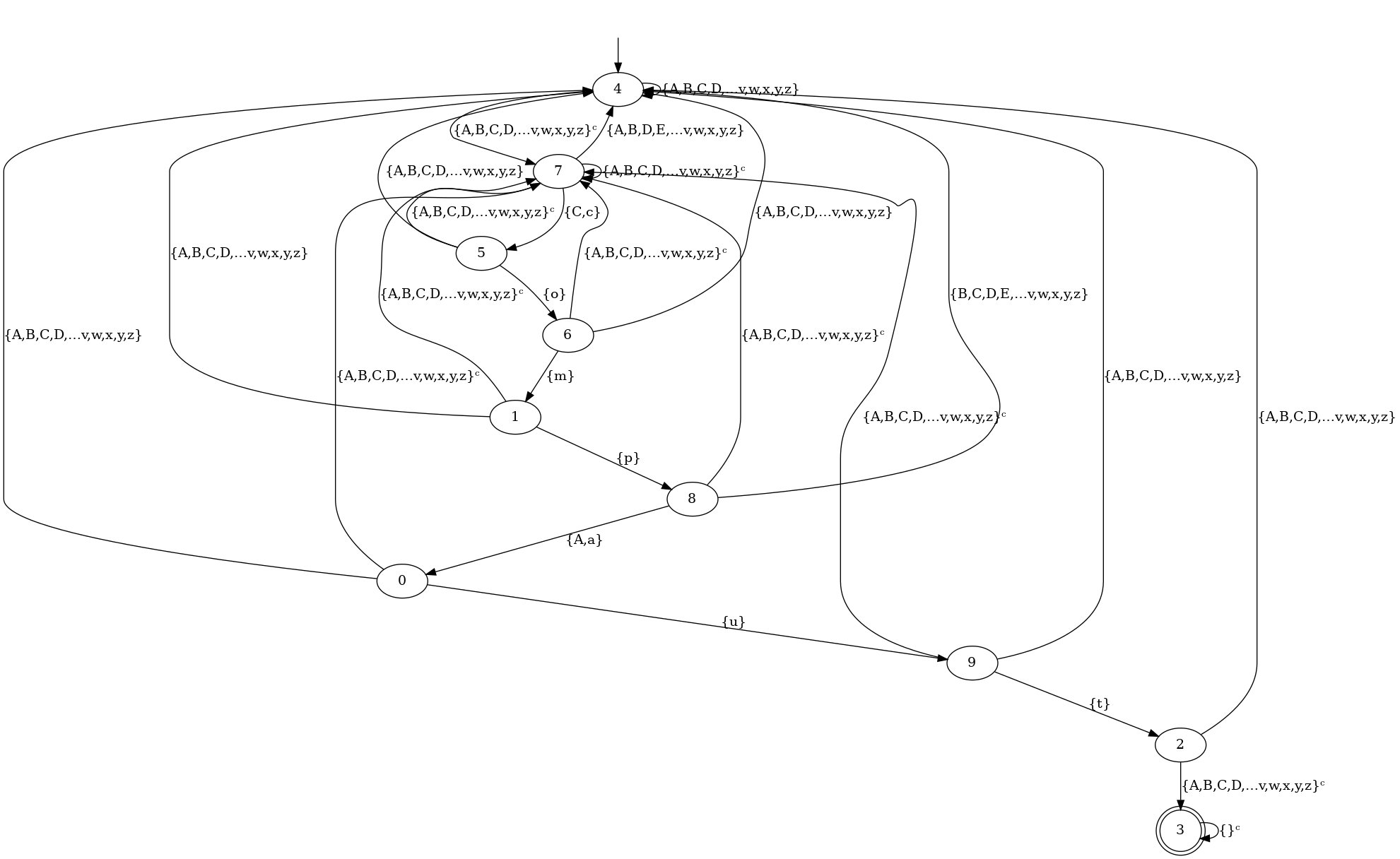In :
import compAut as ca
import sys
from random import choices
from pygments import highlight
from pygments.lexers import CLexer
from pygments.formatters import HtmlFormatter
from IPython.core.display import HTML


# Regexp syntax¶

The regexp syntax follows (loosely) PCRE syntax without grouping and backreference. We construct the automaton the obvious way and minimize it.

In :
regexp = ".*[^a-zA-Z][Cc]omp[aA]ut[^a-zA-Z].*"
L = ca.logics.cre.compile(regexp)


It is possible to display thanks to graphviz the automaton:

In :
L.show()

Out:# Internal representation¶

The automaton is simply seen as a (non mutable) tuple of transitions. Transitions are triple (source, set-of-letters, target) except that the letters can represent cofinite set of letters. It form a symbolic representations of the transitions of an automaton over a possibly infinite alphabet.

In :
it = L.iter_transitions()
print(next(it))
print(next(it))

(4, {A,B,C,D,…v,w,x,y,z}, 4)
(4, {A,B,C,D,…v,w,x,y,z}ᶜ, 7)


Here, the set of letters denotes everything which is not C or c. The internal structure is a dict of dict of dict of dict (with the last "of dict" being useless for automaton model but useful for other models of computation)

In :
L._transitions

Out:
{4: {{A,B,C,D,…v,w,x,y,z}: {4: None}, {A,B,C,D,…v,w,x,y,z}ᶜ: {7: None}},
7: {{C,c}: {5: None},
{A,B,D,E,…v,w,x,y,z}: {4: None},
{A,B,C,D,…v,w,x,y,z}ᶜ: {7: None}},
5: {{o}: {6: None},
{A,B,C,D,…v,w,x,y,z}: {4: None},
{A,B,C,D,…v,w,x,y,z}ᶜ: {7: None}},
6: {{m}: {1: None},
{A,B,C,D,…v,w,x,y,z}: {4: None},
{A,B,C,D,…v,w,x,y,z}ᶜ: {7: None}},
1: {{A,B,C,D,…v,w,x,y,z}: {4: None},
{p}: {8: None},
{A,B,C,D,…v,w,x,y,z}ᶜ: {7: None}},
8: {{B,C,D,E,…v,w,x,y,z}: {4: None},
{A,a}: {0: None},
{A,B,C,D,…v,w,x,y,z}ᶜ: {7: None}},
0: {{A,B,C,D,…v,w,x,y,z}: {4: None},
{u}: {9: None},
{A,B,C,D,…v,w,x,y,z}ᶜ: {7: None}},
9: {{A,B,C,D,…v,w,x,y,z}: {4: None},
{t}: {2: None},
{A,B,C,D,…v,w,x,y,z}ᶜ: {7: None}},
2: {{A,B,C,D,…v,w,x,y,z}: {4: None}, {A,B,C,D,…v,w,x,y,z}ᶜ: {3: None}},
3: {{}ᶜ: {3: None}}}

# Automaton as a model of computation¶

One can execute the automaton over some iterables with the method is_accepted

In :
L.is_accepted("foo CompAut bar")

Out:
True

Nothing is assumed over the alphabet, hence we can feed arbitrary sequences of arbitrary Python object:

In :
L.is_accepted((1, 2)+tuple("compaut")+(object(),))

Out:
True

The automaton represents the regular language it computes. Hence, it inherits the set ability:

In :
"foo CompAut bar" in L

Out:
True
In :
"foo compAut bar" in L

Out:
True
In :
"foo ComppAut bar" in L

Out:
False
In :
("c", "o", "m", "p", "a", "u", "t") in L

Out:
False

# Automaton operations¶

The automaton model support all classical regular operations. Each operation produce a new automaton (nothing is in place).

In :
K = L.concatenate(L)

In :
K.show()

Out: